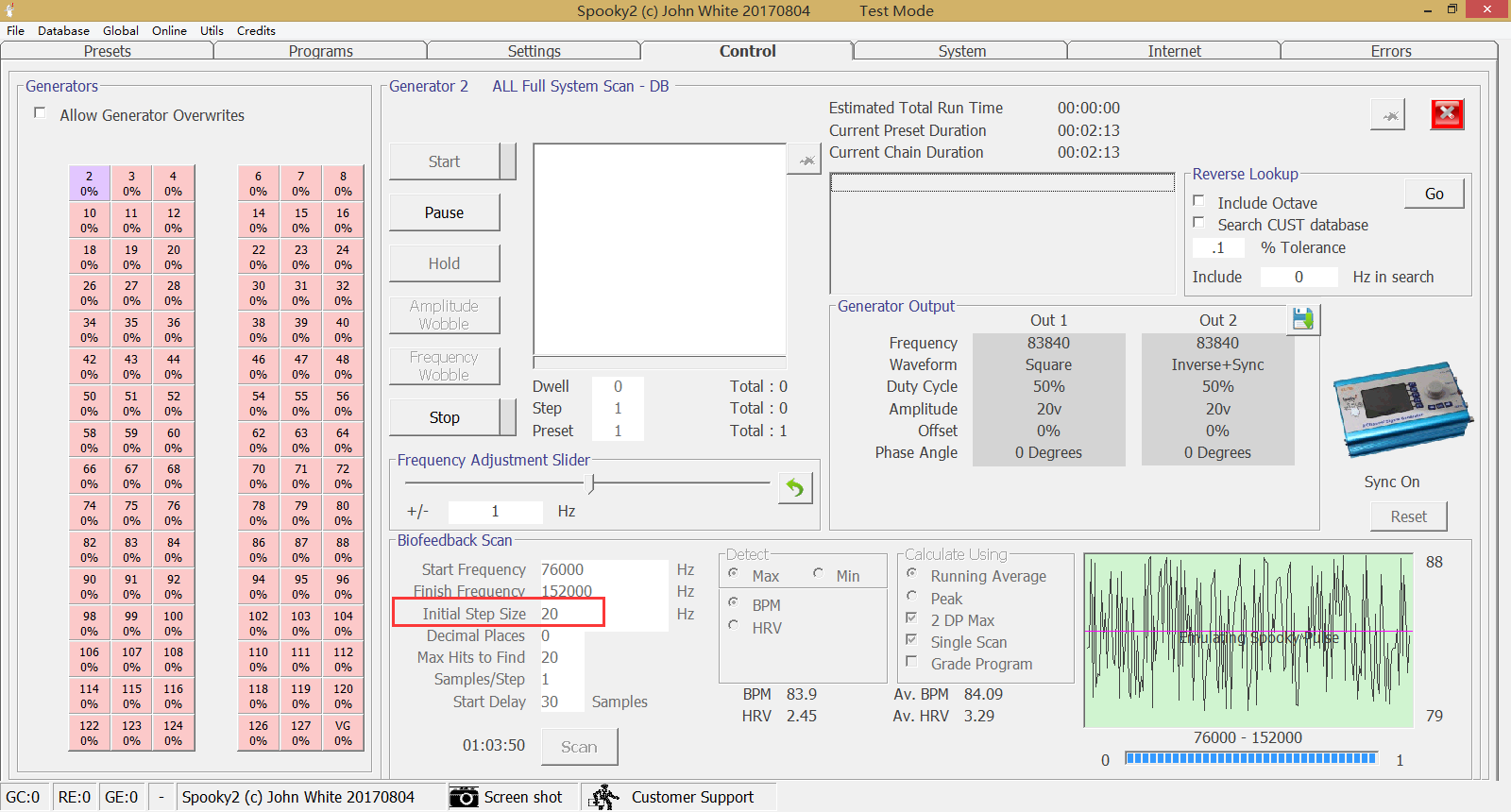# How to Calculate Initial Step Size to Avoid Having Holes in Your Scan 2

The following is to calculate overlapping frequencies by MOR % tolerance. For an octal scan, you will almost always end up with nearly 3800 frequencies to scan in order not to have holes.

With lower frequencies, the range is smaller for an octal range. If you go past this, then you’ll have more frequencies to scan, thus a longer scan time.

Step size can be calculated as follows (different depending on your frequency range).

1. Take the starting frequency which has the smallest resonate range, and do the following:

x / 100 * 0.025

2. Take the result which is the +- range each frequency in your scan is guaranteed to cover.

3. Decide on the step size to use, that incorporates this value as an overlap, and results in an even number of frequencies in the range you are scanning.

Math is fun right?

Example:

76000 – 152000 – Default Scan Range

76000 / 100 * 0.025 = 19 So every frequency above 76000 will have at minimum a +- 19 Hz resonance.

152000 – 76000 = 76000 This is the range of frequencies we are scanning.

76000 / 19 = 4000 So we could use a step size of 19. We use 20 which divides 76000 into 3800 distinct steps.

Each frequency overlaps by 18 Hz.

76000 reaches up to 76019 and 76020 reaches down to 76001.

I know this is not simple, but hopefully the concept can be followed.Authored by Jeff Kaczor
1.1.